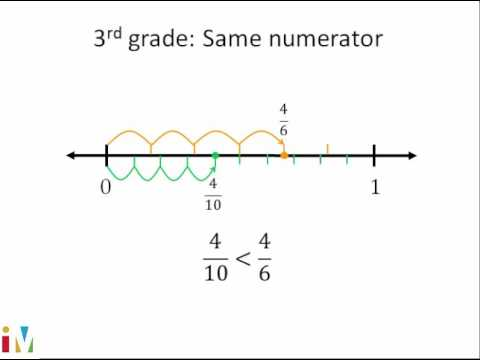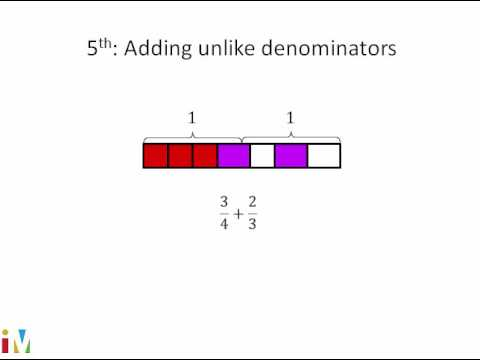Video

# Whole numbers as fractions (Full video)

Description: Use fraction models and a number line to represent 3 as a fraction. Created by Sal Khan. Let's say that this circle right over here represents one whole. And we've divided this circle into one, two, three, four, five equal sections. So each of these sections represents 1/5 of the circle.

### Other videos you might be interested in### Comparing Fractions

#### Illustrative Mathematics